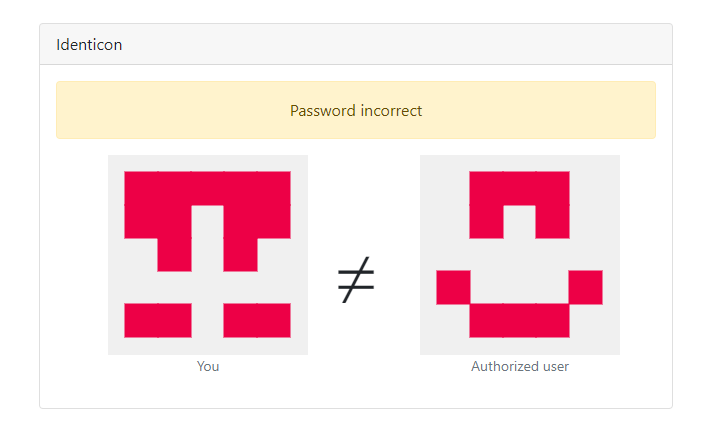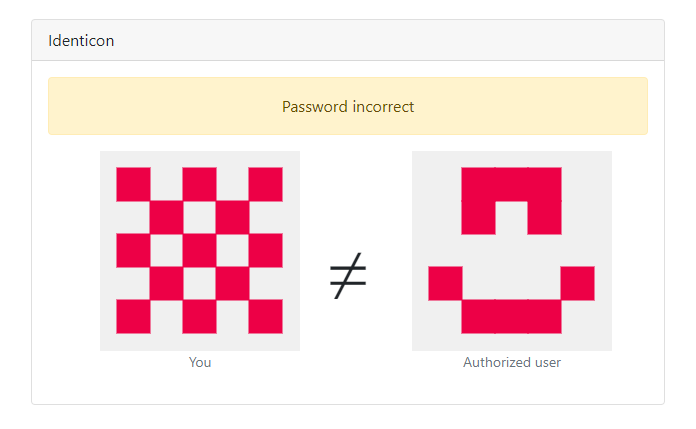WebReversingEncoding +150 points

10 months ago - 91 views

# Identicon

This was a web challenge with source code to the application, which I find really fun. It required some cool reversing of the code to understand what it was exactly doing. In the end, a very satisfying challenge to solve.

## The Challenge

When we start the application, we are prompted with a simple password input. We need to input a password 16 or more characters long. If we submit something random we can see the password is incorrect:On this page, we also see two identicons. From past experience, I remember something like this. An identicon like this is used in Github for example when you create an account, and these are normally generated from a random string of characters.

We also get the source code to the application in a `server.js` file:

JavaScript

``````const Identicon = require('identicon.js') // https://github.com/stewartlord/identicon.js
const express = require('express') // http://expressjs.com/
const bodyParser = require('body-parser');
require('dotenv').config();
const flag = process.env.FLAG || 'nottherealflag'

const valid_user = 'PHN...nPg'  // Long base64 string

const options = {
size: 200,
format: 'svg',
foreground: [237, 0, 70, 255]
}

const app = express()
const port = 3000
app.use(bodyParser.urlencoded({ extended: false }));
app.set('view engine', 'pug')

app.get('/', (req, res) => {
res.render('form')
})

app.post('/', (req, res) => {
// Longer is safer, right?
return res.render('form', { error: 'Password too short (16+ char)' })
}

let user_data = new Identicon(Buffer.from(password).toString('hex'), options).toString();

if (user_data == valid_user) {
res.render('flag', { user: user_data, admin: valid_user, flag: flag })
} else {
res.render('auth_error', { user: user_data, admin: valid_user })
}
})

app.listen(port, () => {
console.log(`Example app listening on port \${port}`)
})``````

Here we can see what's happening. If we GET the home page, we just get returned the form. If we POST the form, we get into the password check. It first generates an identicon with our password and then compares it to the `valid_user` variable. This was a long base64 string, so we might need to reverse it to get back the password it is generated from. Lastly, we get the flag if the password is correct.

## Reversing the Algorithm

Now we should look into how the identicon gets generated because we only see an `Identicon()` function call. At the top, we can see the `identicon.js` library is used though, together with a direct Github link to the repository. In the repository, there is a `package.json` file which is always required for any JavaScript module. It's a good place to check first.

JSON

``````{
"name": "identicon.js",
"version": "2.3.3",
"description": "GitHub-style identicons as PNGs or SVGs in JS.",
"main": "identicon.js",
...
"author": "stewardlord",
}``````

Here the `main` is defined as `identicon.js`. This file then contains the code that is run. When we call the `new Identicon()` function it runs a small piece of code that saves some options of the identicon. Including the `hash`, which is directly the input we give the function. This will be useful for later.

JavaScript

``````var Identicon = function(hash, options){
...
this.hash = hash
...
};``````

We previously found that the `toString()` method is called on the `Identicon` object, so let's take a look at that function in the `identicon.js` file

JavaScript

``````toString: function(raw) {
// backward compatibility with old toString, default to base64
if (raw) {
return this.render().getDump();
} else {
return this.render().getBase64();
}
},``````

Looks like it just calls the `render()` method and encodes it in base64. If we look at this `render()` method it contains the real algorithm:

JavaScript

``````render: function() {
var image      = this.image(),
size       = this.size,
baseMargin = Math.floor(size * this.margin),
cell       = Math.floor((size - (baseMargin * 2)) / 5),
margin     = Math.floor((size - cell * 5) / 2),
bg         = image.color.apply(image, this.background),
fg         = image.color.apply(image, this.foreground);

// the first 15 characters of the hash control the pixels (even/odd)
// they are drawn down the middle first, then mirrored outwards
var i, color;
for (i = 0; i < 15; i++) {
color = parseInt(this.hash.charAt(i), 16) % 2 ? bg : fg;
if (i < 5) {
this.rectangle(2 * cell + margin, i * cell + margin, cell, cell, color, image);
} else if (i < 10) {
this.rectangle(1 * cell + margin, (i - 5) * cell + margin, cell, cell, color, image);
this.rectangle(3 * cell + margin, (i - 5) * cell + margin, cell, cell, color, image);
} else if (i < 15) {
this.rectangle(0 * cell + margin, (i - 10) * cell + margin, cell, cell, color, image);
this.rectangle(4 * cell + margin, (i - 10) * cell + margin, cell, cell, color, image);
}
}

return image;
},``````

This code might look daunting at first, but it's actually pretty simple. There is also a nice comment helping us out:

the first 15 characters of the hash control the pixels (even/odd)
they are drawn down the middle first, then mirrored outwards

If we look at the code we can see this as well. The first 15 characters of the hash are used to determine the color of the pixels. Based on the character value it is converted from a hexadecimal number (16) to an integer. This number determines whether the `bg` (background) or `fg` (foreground) color is used. This is done with the `% 2` which means modulo 2 and is pretty much the remainder after division by 2. An even number would have a remainder of 0, and an odd number would have a remainder of 1. This makes it so the color is `bg` if the number is odd, and `fg` if the number is even.

The rest of the code just places the pixel in the right spot. Like the comment says it just draws from the middle down, and then goes outwards mirroring the icon. To make it more clear this would be the order:

Ìdenticon

``````|b|6|1|6|b|
|c|7|2|7|c|
|d|8|3|8|d|
|e|9|4|9|e|
|f|a|5|a|f|``````

## Creating our own Identicon

Now that we understand the algorithm in the `identicon.js` library, let's look back at the challenge. The following line generates our identicon:

JavaScript

``let user_data = new Identicon(Buffer.from(password).toString('hex'), options).toString();``

So our password from the input gets converted to a hexadecimal string, and then passed to the `new Identicon()` function. Finally, it renders the identicon with the `toString()` method.

But the `identicon.js` library expects a hash, not just raw hex input. These are both hexadecimal strings, but in our case, we have full control of the string, where it would normally be random with a hash.

We learned from the reversing that the function just goes through all the characters of the hash one by one, and uses the `% 2` to determine the color. So if we input some string like `AAAAAAAAAAAAAAAA` (16 a's), it gets converted to `4141414141414141...`. Then for every character, it checks if it is even or odd. The `4` is even, so it uses the `fg` color, and the `1` is odd, so it uses the `bg` color. This means we should get an alternating color pattern. Testing this in the application this is indeed correct:We can now just make our own hex string that will turn into the authorized user's identicon. We can use a `4` for an even (`fg`) color, and `5` for an odd (`bg`) color. We just need to follow the pattern in the order above, from the authorized user and make the following string:

``455544455455545``

This is still in hexadecimal, so we need to convert it to ASCII to input it as a password. It is also not long enough yet because only the first 15 characters are used. This means it doesn't matter what characters come after, so we can just add some random hex characters to the end to fill the space.

Converting this to hex with a CyberChef recipe we get a password like this:

``EUDETUTVffffffff``

The first characters are the identicon, and the `f`'s are just to fill the space. If we now try this password in the input it should generate the correct identicon and give us the flag. And sure enough, we got through the password check and see the flag!
`CTF{482c811da5d5b4bc6d497ffa98491e38}`